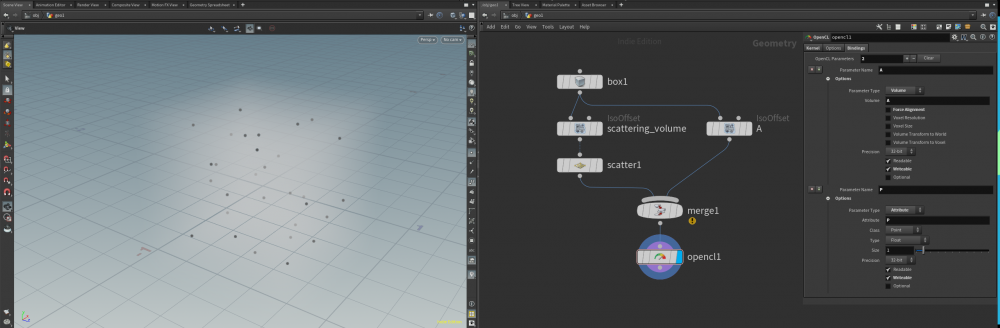# Accessing the nearest voxel from a point in OpenCL node

## Recommended Posts

Hello, I'm trying to implement an OpenCL node that loops over "scatter1" node's points and changes density at the volume A's nearest voxel of every point.

However, I can't figure out how to calculate the nearest voxel's index value.

So I'd like to know how to calculate the voxel's index value.

Also, in the "merge1" node, there is one point added by the volume A(Points count is increased by one after merging).

Is there a way to exclude that point when looping over points?

I attached my node setup and "opencl1" node's bindings setup below.

Thank you.## Create an account

Register a new account